# 详解编辑距离（Levenshtein 距离）算法

## 目标

72. 编辑距离

• 插入一个字符

• 删除一个字符

• 替换一个字符

输入：word1 = "horse", word2 = "ros"

horse -> rorse (将 'h' 替换为 'r')
rorse -> rose (删除 'r')
rose -> ros (删除 'e')


## 概念铺垫

【定义】 $f(i,j)$ 表示将 w[i] 代表串，变成 w[j] 代表串所需的最小步骤数。

DP 表的解释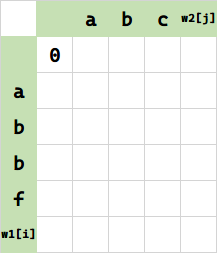## 初始化 DP 数组，尝试寻找规律

$f(0,0)$ ，将 (空串) 变成 (空串) 需要 0 步，无脑填 0.$f(0,1)$，将空串变 a 需要一步插入，填 1.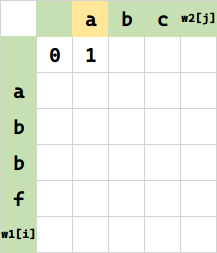f(0,2)，将空串变 ab 需要两步插入，填 2. 相当于 $f(0,1) + 1$。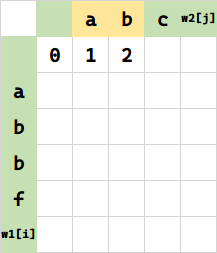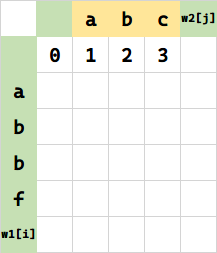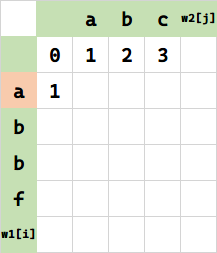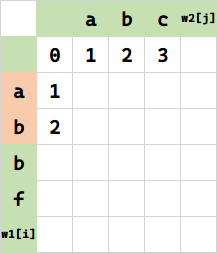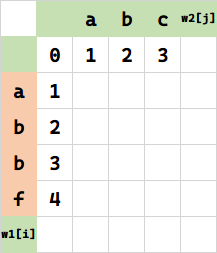## 归纳：如何利用历史状态计算新状态？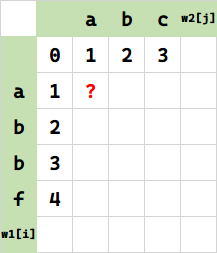（请时刻关注上面这个求职目标的位置）

### 插入的情况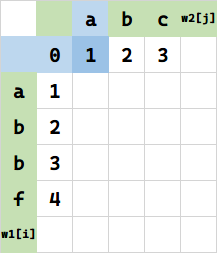f(0,1) 表示 w1[i-1] 代表串变成 w2[i] 代表串 所需次数，查表得到 $1$。

1 表示 w1[i-1] 代表串 之后再插入一个 a 这一次动作的计数。

### 替换的情况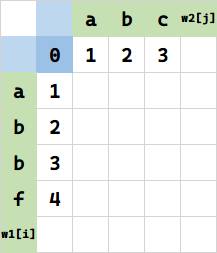### 删除的情况（等价于插入）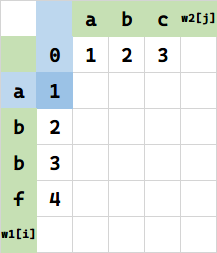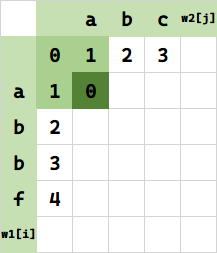$c_2$ 是替换操作，w1[i] == w2[i] 即字符相同时不用替换，开销是 0，不同时替换，开销是 1.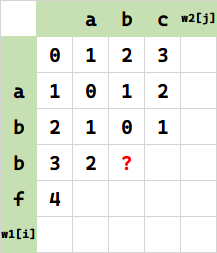• 怎么求 $C_1$ ？让 w1[i-1] 代表串 ab  变成 w2[j] 代表串 ab 的开销是 0，要经过一步插入，所以 $c_1 =1$，$C_1 = 1+1=2$

• 同理让 w1[i] 代表串 abb 变成 w2[j-1] 代表串 a  的开销是 2（上表 $f(i,j-1)$），然后要经过一步删除，所以 $c_3 =1$，$C_3 = 2+1=3$

• 再看 $C_2$，由于 $f(i-1,j-1)$ 即让 w1[i-1] 代表串（ab）变成 w2[j-1] 代表串（a）的开销是 $f(i-1,j-1) = 1$，而 w1[i]==w2[j]，所以 $c_2 = 0$，故 $C_2 = 1+0 = 1$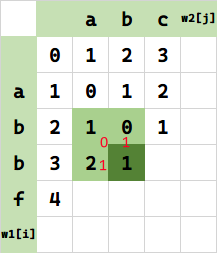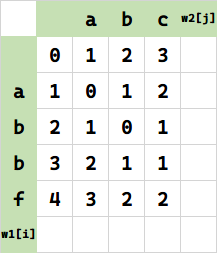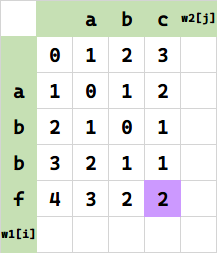## 用代码表达出来

 1inline int min3(int a, int b, int c) {
2  return a < b ? (c < a ? c : a) : (b > c ? c : b);
3}
4
5class Solution {
6public:
7  int minDistance(string w1, string w2) {
8    int n = w1.size();
9    int m = w2.size();
10    vector<vector<int>> dp(n + 1, vector<int>(m + 1, 0));
11    // -- 初始化基本情况
12    // +-------> y
13    // |
14    // x
15    //
16    // 第一列的各行
17    for (int i = 1; i <= n; i++) {
18      dp[i] = i;
19    }
20    // 第一行的各列
21    for (int j = 1; j <= m; j++) {
22      dp[j] = j;
23    }
24    // -- 开始 DP
25    for (int i = 1; i <= n; i++) {   // 各行
26      for (int j = 1; j <= m; j++) { // 各列
27        auto c1 = dp[i - 1][j] + 1;  // 上
28        auto cc2 = w1[i - 1] == w2[j - 1] ? 0 : 1;
29        auto c2 = dp[i - 1][j - 1] + cc2; // 左上
30        auto c3 = dp[i][j - 1] + 1;   // 左
31        dp[i][j] = min3(c1, c2, c3);
32      }
33    }
34
35    return dp[n][m];
36  }
37};


## 空间优化

$f(i,j)$ 只依赖当前行和前一行。但是初始化的时候却要初始化最左列全部。如果优化为 $O(m)$ 空间，势必要破坏最左列的初始化。但是注意到初始化的结构和遍历的结构很像，只要把“第一列的各行”初始化放到后面就行：

 1class Solution {
2public:
3  int minDistance(string w1, string w2) {
4    int n = w1.size();
5    int m = w2.size();
6    int d = 2;
7    vector<vector<int>> dp(d, vector<int>(m + 1, 0));
8    // 第一行的各列
9    for (int j = 1; j <= m; j++) {
10      dp[j] = j;
11    }
12    // -- 开始 DP
13    for (int i = 1; i <= n; i++) { // 各行
14      dp[i % d] = i; // 初始化移动到这儿了
15      for (int j = 1; j <= m; j++) {      // 各列
16        auto c1 = dp[(i - 1) % d][j] + 1; // 上
17        auto cc2 = w1[i - 1] == w2[j - 1] ? 0 : 1;
18        auto c2 = dp[(i - 1) % 2][j - 1] + cc2; // 左上
19        auto c3 = dp[i % d][j - 1] + 1;
20        dp[i % d][j] = min3(c1, c2, c3);
21        // print_vec_2d(dp);
22      }
23    }
24
25    // print_vec_2d(dp);
26
27    return dp[n % 2][m];
28  }
29};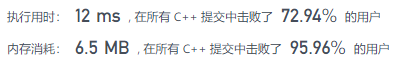## 进一步空间优化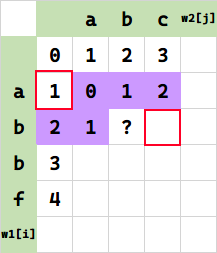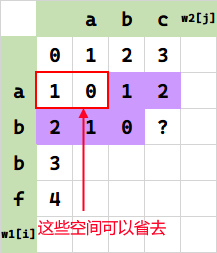1class Solution {
2public:
3  int minDistance(string w1, string w2) {
4    int n = w1.size();
5    int m = w2.size();
6    if (n == 0)
7      return m;
8    if (m == 0)
9      return n;
10    std::vector<int> dp(m + 1);
11    for (int c = 0; c < m + 1; c++) {
12      dp[c] = c;
13    }
14    for (int r = 1; r < n + 1; r++) {
15      int pre = r;
16      for (int c = 1; c < m + 1; c++) {
17        int cur = min(dp[c], pre) + 1;
18        if (w1[r - 1] == w2[c - 1]) {
19          cur = min(cur, dp[c - 1]);
20        } else {
21          cur = min(cur, dp[c - 1] + 1);
22        }
23        dp[c - 1] = pre;
24        pre = cur;
25        if (c == m)
26          dp[c] = cur;
27      }
28    }
29    return dp[m];
30  }
31};